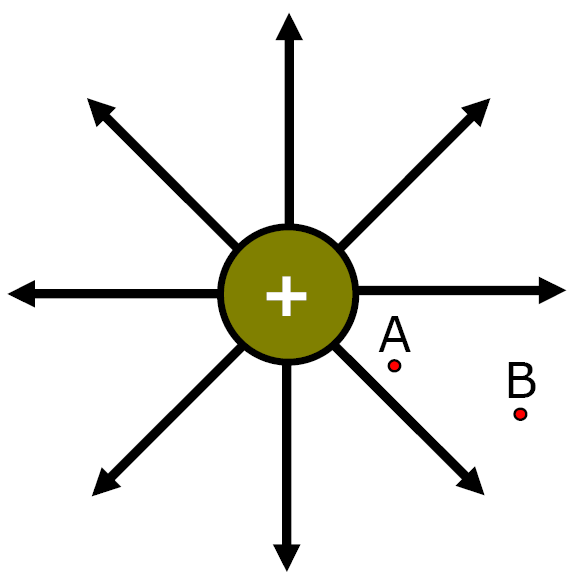Physics Fun with Gum

(Seem to need a large stick of gun to make this work.)

I.) Electric Potential - work needed or energy acquired when a positive charge is moved from infinity to a certain point in an electric field.II) Potential Difference (Voltage) potential energy difference between 2 points in an electric field per unit of chargeA) Potential Difference, Energy & Work

As a charge moves through an electric field it gains PE or KEIf "Opposites" move

CloserGain KE or PE?

They Gain KE'Opposites' Separate

Gain KE or PE?

They Gain PEIf "Likes" SeparateGain KE or PE?

They Gain KEIf 'Likes' move...

closer

Gain KE or PE?

They Gain PE1) Equation #1

 V = W (Joules) q (Coulombs)

W = work done against field or energy acquired working with field (Joules or eV)

eV = electronVolts

(small energy unit)

q - amount of charge moving through field (Coulombs)

V - Potential Difference (volts)

 Ex) It takes 6 J of work to move 2 C of charge between 2 points in an electric field. What is the potential energy difference (V) between these points?

Ex) It takes 6 Joules of work to move 2 coulombs of charge between 2 points in an electric field. What is the potential energy difference (voltage) between these 2 points?

 V = W (Joules) q (Coulombs)

 V = 6 Joules 2 Coulombs

V = 3 Volts or J/C

2) Potential difference (V) #2

 V = W (eV) q (elementary charges)

W = work done against field or energy acquired working with field

(Electronvolts = Ev)

Electronvolt
– small energy unit = 1.6 x 10-19 J

q - amount of charge moving through field (# elementary charges)

V - Potential Difference (volts)

 Ex) It takes 10 eV to move 2 elementary charges from one point to another in an electric field. Find the potential difference between these points.

Ex) It takes 10 eV to move 2 elementary charges from one point to another in an electric field. Find the potential difference between these points.

 V = W (eV) q (elementary charges)

 V = 10 eV 2 elementary charges

V = 5 Volts

 Ex) +2 elementary charges acquire 16 joules of energy when they move between 2 points in an electric field. What is the potential difference (voltage) between these two points in the field?

Ex) +2 elementary charges acquire 16 joules of energy when they move between 2 points in an electric field. What is the potential difference (voltage) between these two points in the field?

Units don't match!!!

elementary charges, eV   Joules/Coulombs

+2 elementary charges=

3.2 x 10-19 C

 V = W (Joules) q (Coulombs)

 V = 16 J (3.2 x 10-19 C)

Calculator Review

 Scientific Notationn TI83Plus

V = 5.0 x 1019 Volts

or J/C

 Ex) If 4.8 x 10-17 joules of work is required to move an electron between 2 points in an electric field, what is the electric potential difference between these points?a) 3.0 x 102 V b) 4.8 x 10-17 V c) 1.6 x 10-19 V d) 4.8 x 102 V

 Ex) If 4.8 x 10-17 joule of work is required to move an electron between 2 points in an electric field, what is the electric potential difference between these points?a) 3.0 x 102 V b) 4.8 x 10-17 V c) 1.6 x 10-19 V d) 4.8 x 102 V

W = 4.8 x 10-17 Joule

V = ?

q = ?

Hint: The charge in this problem is an electron

q = 1.6 x 10-19 C

 V = W (Joules) q (Coulombs)

V = 4.8 x 10-17 J / (1.6 x 10-19)C

a) 3.0 x 102 V